# PSAT Math : How to find domain and range of the inverse of a relation

## Example Questions

### Example Question #1 : How To Find Domain And Range Of The Inverse Of A Relation

What is the range of the function y = x2 + 2?

{–2, 2}

all real numbers

undefined

{2}

y ≥ 2

y ≥ 2

Explanation:

The range of a function is the set of y-values that a function can take. First let's find the domain. The domain is the set of x-values that the function can take. Here the domain is all real numbers because no x-value will make this function undefined. (Dividing by 0 is an example of an operation that would make the function undefined.)

So if any value of x can be plugged into y = x2 + 2, can y take any value also? Not quite! The smallest value that y can ever be is 2. No matter what value of x is plugged in, y = x2 + 2 will never produce a number less than 2. Therefore the range is y ≥ 2.

### Example Question #1 : How To Find Domain And Range Of The Inverse Of A Relation

Which of the following values of x is not in the domain of the function y = (2x – 1) / (x2 – 6x + 9) ?

–1/2

1/2

2

3

0

3

Explanation:

Values of x that make the denominator equal zero are not included in the domain. The denominator can be simplified to (x – 3)2, so the value that makes it zero is 3.

### Example Question #1 : How To Find Domain And Range Of The Inverse Of A Relation

Given the relation below:

{(1, 2), (3, 4), (5, 6), (7, 8)}

Find the range of the inverse of the relation.

{1, 3, 5, 7}
{2, 4, 6, 8}
{1, 2, 3, 4}
{5, 6, 7, 8}
the inverse of the relation does not exist
Correct answer: {1, 3, 5, 7}
Explanation:

The domain of a relation is the same as the range of the inverse of the relation.  In other words, the x-values of the relation are the y-values of the inverse.

### Example Question #1 : How To Find Domain And Range Of The Inverse Of A Relation

What is the range of the function y = x2 + 2?

all real numbers

undefined

{–2, 2}

y ≥ 2

{2}

y ≥ 2

Explanation:

The range of a function is the set of y-values that a function can take. First let's find the domain. The domain is the set of x-values that the function can take. Here the domain is all real numbers because no x-value will make this function undefined. (Dividing by 0 is an example of an operation that would make the function undefined.)

So if any value of x can be plugged into y = x2 + 2, can y take any value also? Not quite! The smallest value that y can ever be is 2. No matter what value of x is plugged in, y = x2 + 2 will never produce a number less than 2. Therefore the range is y ≥ 2.

### Example Question #2 : How To Find Domain And Range Of The Inverse Of A Relation

What is the smallest value that belongs to the range of the function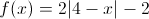?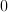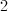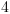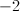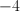Explanation:

We need to be careful here not to confuse the domain and range of a function. The problem specifically concerns the range of the function, which is the set of possible numbers of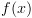. It can be helpful to think of the range as all the possible y-values we could have on the points on the graph of.

Notice thathas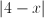in its equation. Whenever we have an absolute value of some quantity, the result will always be equal to or greater than zero. In other words, |4-x|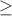0. We are asked to find the smallest value in the range of, so let's consider the smallest value of, which would have to be zero. Let's see what would happen toif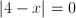.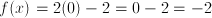This means that when,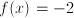. Let's see what happens whengets larger. For example, let's let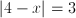.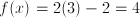As we can see, asgets larger, so does. We wantto be as small as possible, so we are going to wantto be equal to zero. And, as we already determiend,equalswhen.

The answer is.

### Example Question #1 : How To Find Domain And Range Of The Inverse Of A Relation

If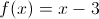, then find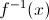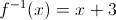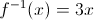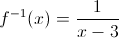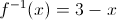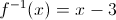Explanation:is the same as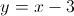To find the inverse simply exchangeand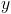and solve forSo we get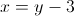which leads to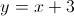.

### Example Question #1 : How To Find Domain And Range Of The Inverse Of A Relation

If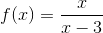, then which of the following is equal to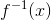?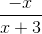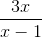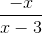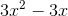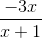Explanation: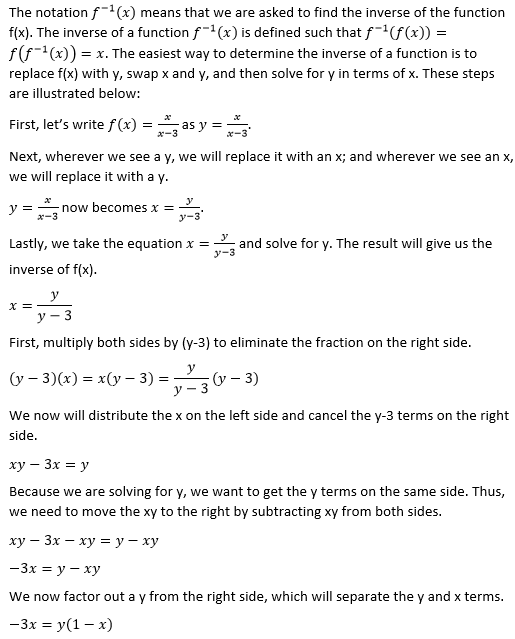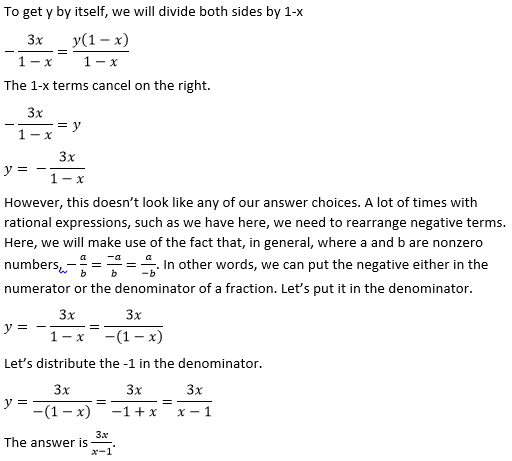### Example Question #7 : How To Find Domain And Range Of The Inverse Of A Relation

Given the relation below, identify the domain of the inverse of the relation.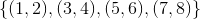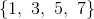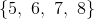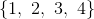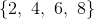The inverse of the relation does not exist.Explanation:The domain of the inverse of a relation is the same as the range of the original relation. In other words, the y-values of the relation are the x-values of the inverse.

For the original relation, the range is:.

Thus, the domain for the inverse relation will also be.

### All PSAT Math Resources